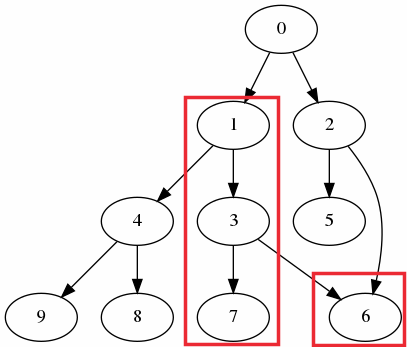In this quick article, we'll have a look at how to list all parent/child nodes in Pandas DataFrame. This might be useful in certain scenarios like verifying trees or networks.

## Step 1: Prepare Hierarchical data

Lets prepare hierarchical data which is going to be used for our example:

``````import pandas as pd

df = pd.DataFrame({
'parent': [0, 0, 1, 1, 2, 2, 3, 3, 4, 4],
'child': [1, 2, 3, 4, 5, 6, 6, 7, 8, 9]
})
``````

Sometimes parent or child information might be stored in the index. In those cases index can be transfered to a column by:

``````df_req['index1'] = df_req.index
``````

## Step 2: Install Python package networkx

As a second step we need to install package: `networkx` by:

``````pip install networkx
``````

This package is used for creation, manipulation, and analysis of the structure and features of complex networks.

## Step 3: Listing Subtree Descendants with NetworkX in Pandas DataFrame

Finally if we like to get all descendants of node 1 in this DataFrame we can do it by converting the DataFrame records to NetworkX nodes:

``````import networkx as nx

g=nx.DiGraph()
``````

and then listing the subtree of NetworkX by:

``````from networkx.algorithms.traversal.depth_first_search import dfs_tree

x = dfs_tree(g, 1)
x.edges()
``````

Which will result in:

`OutEdgeView([(1, 3), (1, 4), (3, 6), (3, 7), (4, 8), (4, 9)])`

Visually the same can be represented by:## Step 4: Set List of Descendants for each Row(Optional)

In this step we are going to add a new column with a list of all descendants recursively.

``````def get_descendants(parent):
descendants = list(dfs_tree(g, parent).edges())
return [x for x in descendants]

df["descendants"] = df["parent"].apply(get_descendants)
``````

This will create new column with a list of all childs for the current parent:

parent child descendants
0 0 1 [1, 3, 6, 7, 4, 8, 9, 2, 5, 6]
1 0 2 [1, 3, 6, 7, 4, 8, 9, 2, 5, 6]
2 1 3 [3, 6, 7, 4, 8, 9]
3 1 4 [3, 6, 7, 4, 8, 9]
4 2 5 [5, 6]
5 2 6 [5, 6]
6 3 6 [6, 7]
7 3 7 [6, 7]
8 4 8 [8, 9]
9 4 9 [8, 9]

If you like to use custom code version you can use the one below. The code is a bit slower than the previous version:

``````def get_children(parent_id):
list_of_children = []

def dfs(parent_id):
child_ids = df[df["parent"]==parent_id]["child"]
if child_ids.empty:
return
for child_id in child_ids:
list_of_children.append(child_id)
dfs(child_id)

dfs(parent_id)
return list_of_children

df["descendants"] = df["parent"].apply(get_children)
``````